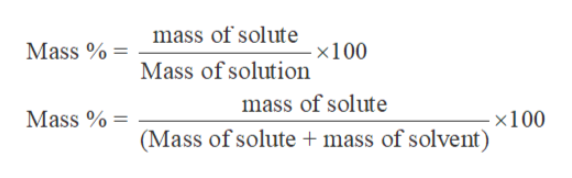# How many grams of a solution that is 5.2% sucrose by mass are needed toobtain 18 g of sucrose.

Question
63 views
How many grams of a solution that is 5.2% sucrose by mass are needed to
obtain 18 g of sucrose.
check_circle

Step 1

Solution stoichiometry involves the calculation of concentration of solutions in the given conditions of volumes, moles etc.

Solution stoichiometry is mainly based on the calculation of moles and volumes. These two values are used to calculate the molarity of solution.

Step 2

Mass percent or weight percent of a solution is the mass of the solute by the mas...help_outlineImage Transcriptionclosemass of solute Mass %= - x100 Mass of solution mass of solute Mass %= - x100 (Mass of solute mass of solvent) fullscreen

### Want to see the full answer?

See Solution

#### Want to see this answer and more?

Solutions are written by subject experts who are available 24/7. Questions are typically answered within 1 hour.*

See Solution
*Response times may vary by subject and question.
Tagged in

### Electrochemistry